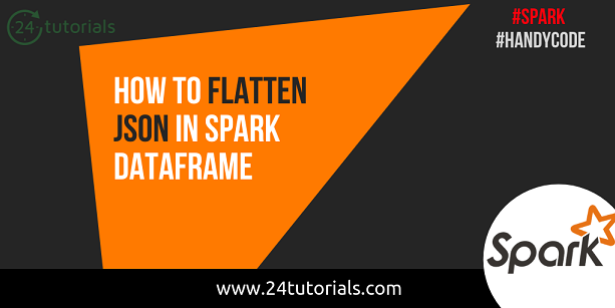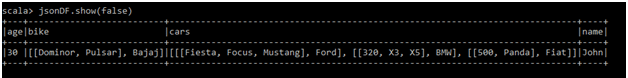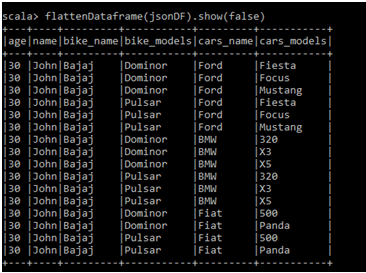Spark

# How to flatten JSON in Spark DataframeHow to flatten whole JSON containing ArrayType and StructType in it?

• In order to flatten a JSON completely we don’t have any predefined function in Spark. We can write our own function that will flatten out JSON completely.
• We will write a function that will accept DataFrame. For each field in the DataFrame we will get the DataType.
1. If the field is of ArrayType we will create new column with exploding the ArrayColumn using Spark explode_outer function.
2. If the field is of StructType we will create new column with parentfield_childfield for each field in the StructType Field.
• This is a recursive function. Once the function doesn’t find any ArrayType or StructType. It will return the flattened DataFrame. Otherwise, It will it iterate through the schema to completely flatten out the JSON.

Sample JSON:
{
“name”:”John”,
“age”:30,
“bike”:{
“name”:”Bajaj”, “models”:[“Dominor”, “Pulsar”]
},
“cars”: [
{ “name”:”Ford”, “models”:[ “Fiesta”, “Focus”, “Mustang” ] },
{ “name”:”BMW”, “models”:[ “320”, “X3”, “X5” ] },
{ “name”:”Fiat”, “models”:[ “500”, “Panda” ] }
]
}

Schema of JSON DataFrame Before Flattening:

```scala> jsonDF.printSchema
root
|-- age: long (nullable = true)
|-- bike: struct (nullable = true)
|    |-- models: array (nullable = true)
|    |    |--element:string(containsNull=true)
|    |-- name: string (nullable = true)
|-- cars: array (nullable = true)
|    |-- element: struct (containsNull = true)
|    |    |-- models: array (nullable = true)
|    |    |    |--element:string(containsNull=true)
|    |    |-- name: string (nullable = true)
|-- name: string (nullable = true)```
• Here we can see that Bike Field is StructType with models and name as child fields in it. When we apply our function over this DataFrame. We will get new fields of bike_models and bike_name. Similary all the fields with StructType will create new fields.
• models field inside the bike field is of ArrayType. This field will be exploded for the values in it. In our case we have two models for Bajaj i.e. Dominor, Pulsar. Exploded column will be like
• Before Exploding
 bike_name bike_models Bajaj [“Dominor”, “Pulsar”]

• After Exploding
 bike_name bike_models Bajaj Dominor Bajaj Pulsar

• Similarly all the fields with ArrayType will be exploded.

Schema of JSON DataFrame After Flattening

```scala> flattenDataframe(jsonDF).printSchema
root
|-- age: long (nullable = true)
|-- name: string (nullable = true)
|-- bike_name: string (nullable = true)
|-- bike_models: string (nullable = true)
|-- cars_name: string (nullable = true)
|-- cars_models: string (nullable = true)
```

DataFrame Before FlatteningDataFrame After FlatteningBelow is the program which does the purpose –